# Displacement and Velocity More Practice Displacement and Velocity

• Slides: 35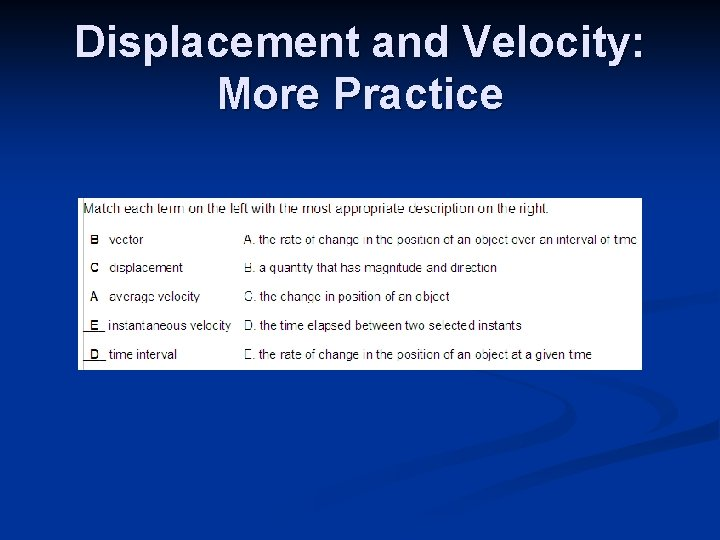Displacement and Velocity: More PracticeDisplacement and Velocity: More Practice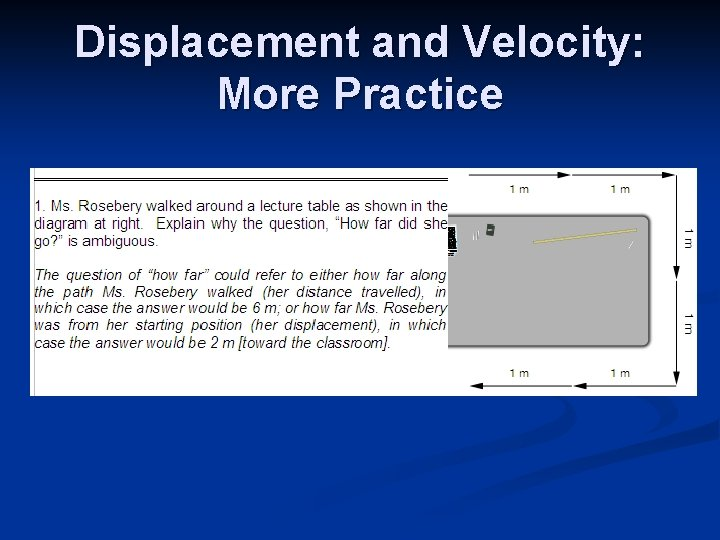Displacement and Velocity: More PracticeDisplacement and Velocity: More PracticeExperimental Uncertainty: Learning Goals The student will be able to formulate scientific questions and hypotheses. (A 1. 1) n The student will be able to identify independent, and controlled variables. (A 1. 5) n The student will be able to identify sources of error and represent errors numerically. (A 1. 8, A 1. 12) nExperiments in Physics SPH 4 C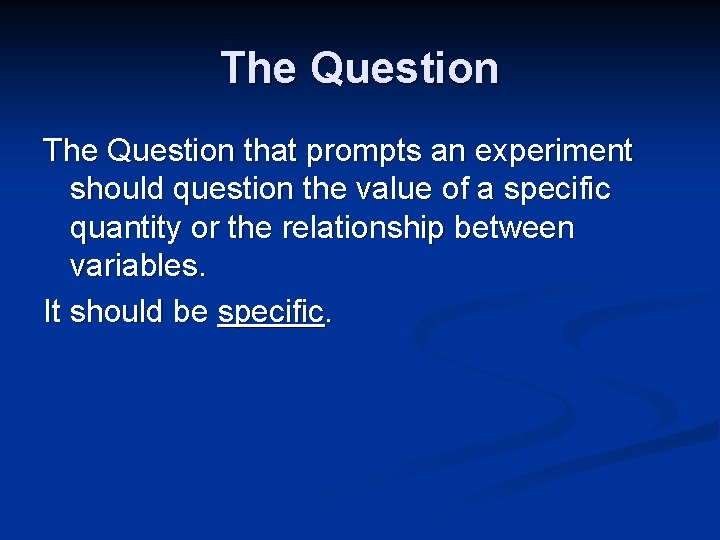The Question that prompts an experiment should question the value of a specific quantity or the relationship between variables. It should be specific.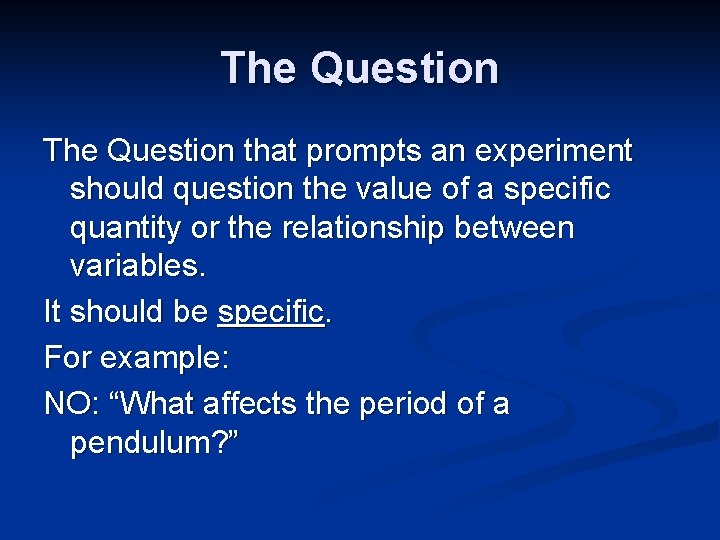The Question that prompts an experiment should question the value of a specific quantity or the relationship between variables. It should be specific. For example: NO: “What affects the period of a pendulum? ”The Question that prompts an experiment should question the value of a specific quantity or the relationship between variables. It should be specific. For example: NO: “What affects the period of a pendulum? ” YES: “What is the period of a 1 -m pendulum? ”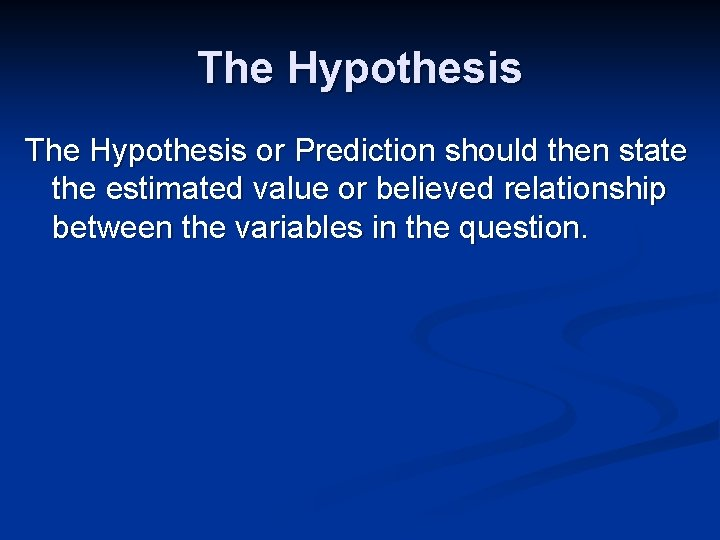The Hypothesis or Prediction should then state the estimated value or believed relationship between the variables in the question.The Hypothesis or Prediction should then state the estimated value or believed relationship between the variables in the question. Question: “What effect does the mass of a pendulum have on the period of the pendulum? ”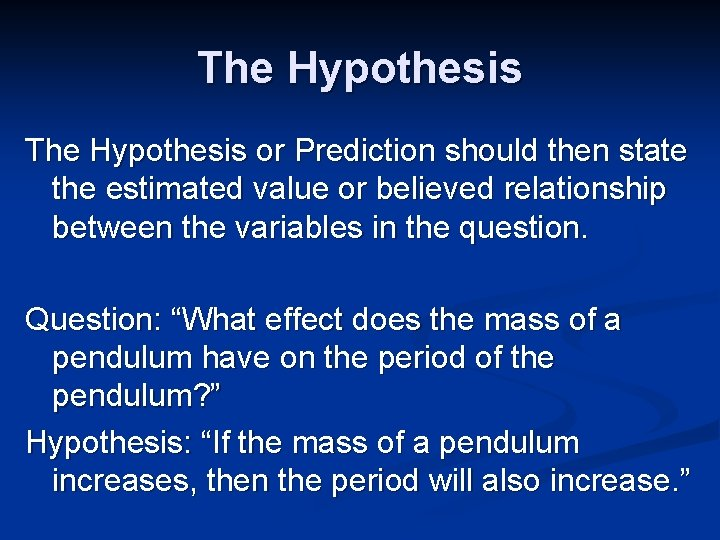The Hypothesis or Prediction should then state the estimated value or believed relationship between the variables in the question. Question: “What effect does the mass of a pendulum have on the period of the pendulum? ” Hypothesis: “If the mass of a pendulum increases, then the period will also increase. ”Variables A variable is anything in an experiment that may be changed. Most experiments change only two: n the independent variable, which is the one changed by the experimenter n the dependent variable, which is the one for which the experimenter measures the response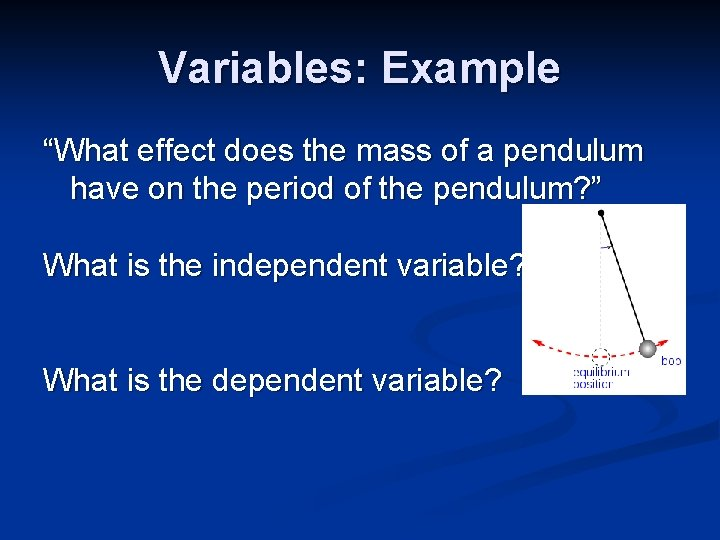Variables: Example “What effect does the mass of a pendulum have on the period of the pendulum? ” What is the independent variable? What is the dependent variable?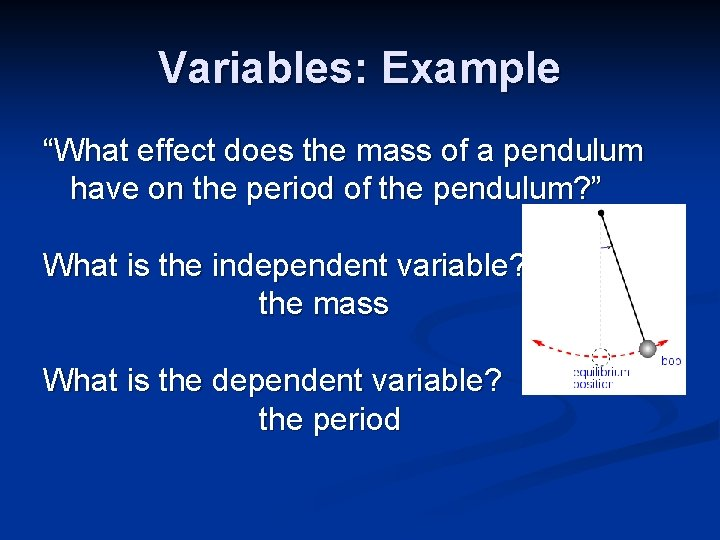Variables: Example “What effect does the mass of a pendulum have on the period of the pendulum? ” What is the independent variable? the mass What is the dependent variable? the period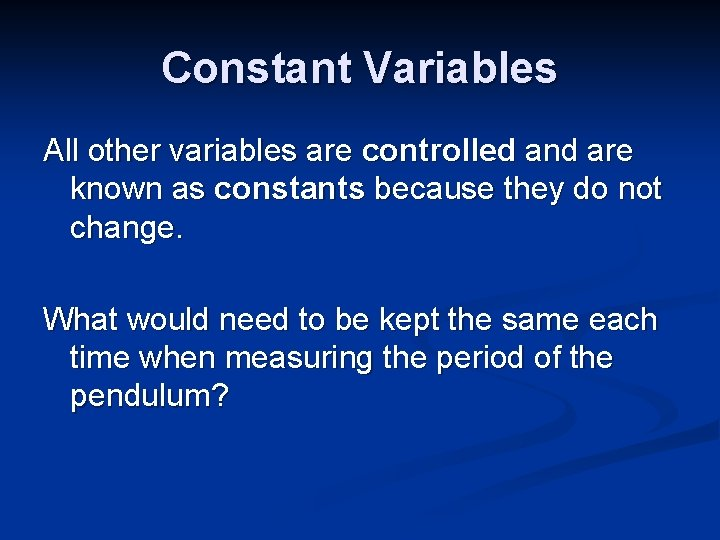Constant Variables All other variables are controlled and are known as constants because they do not change. What would need to be kept the same each time when measuring the period of the pendulum?Constant Variables All other variables are controlled and are known as constants because they do not change. What would need to be kept the same each time when measuring the period of the pendulum? the length of the pendulum the displacement of the pendulum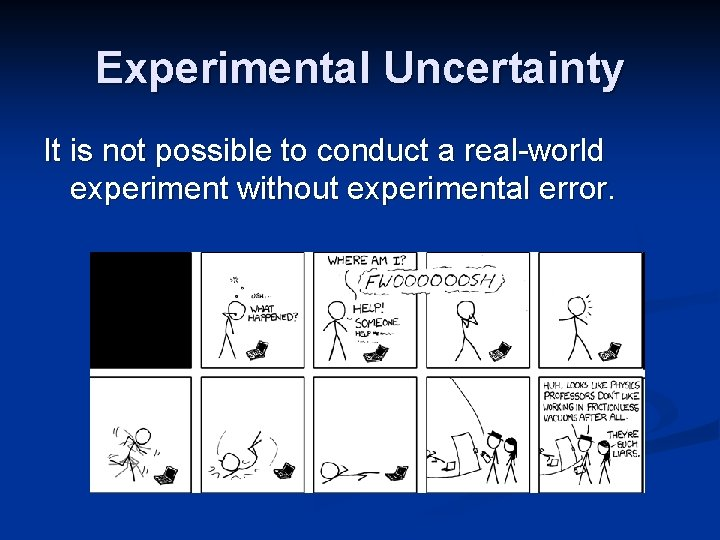Experimental Uncertainty It is not possible to conduct a real-world experiment without experimental error.Experimental Uncertainty It is not possible to conduct a real-world experiment without experimental error. These errors are not mistakes; a better term for them might be experimental uncertainties.Experimental Uncertainty It is not possible to conduct a real-world experiment without experimental error. These errors are not mistakes; a better term for them might be experimental uncertainties, which may be due to either the measuring devices (instrumental uncertainties) or how they were used (procedural uncertainties).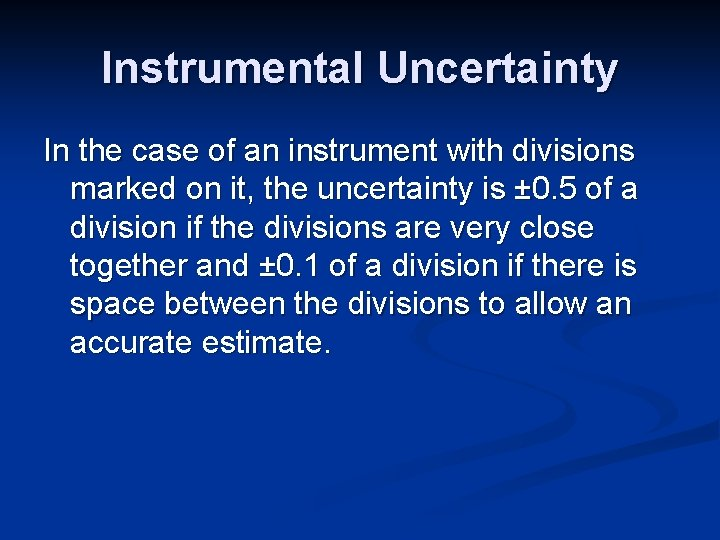Instrumental Uncertainty In the case of an instrument with divisions marked on it, the uncertainty is ± 0. 5 of a division if the divisions are very close together and ± 0. 1 of a division if there is space between the divisions to allow an accurate estimate.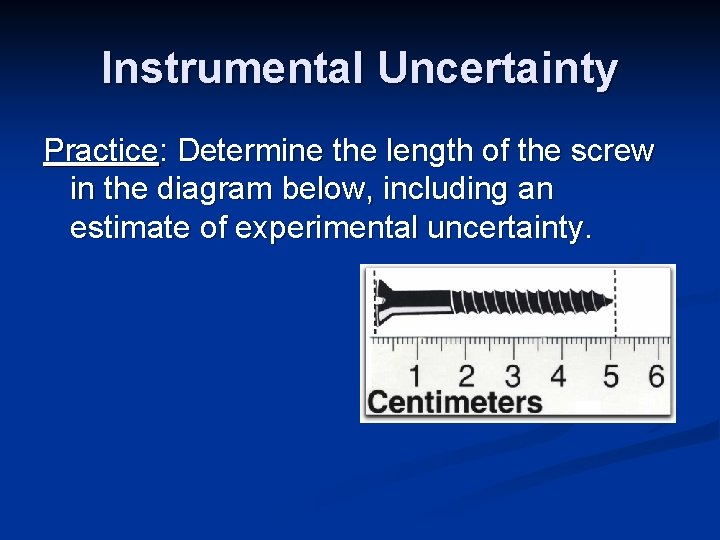Instrumental Uncertainty Practice: Determine the length of the screw in the diagram below, including an estimate of experimental uncertainty.Instrumental Uncertainty Practice: Determine the length of the screw in the diagram below, including an estimate of experimental uncertainty. 5. 10 ± 0. 05 cm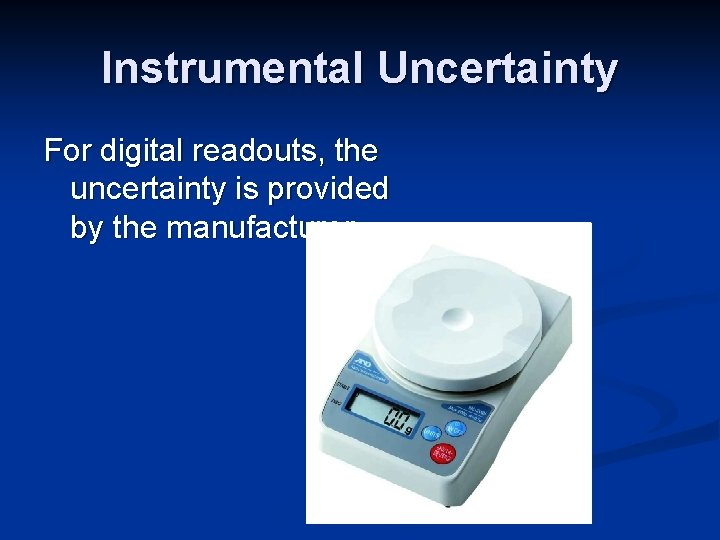Instrumental Uncertainty For digital readouts, the uncertainty is provided by the manufacturer.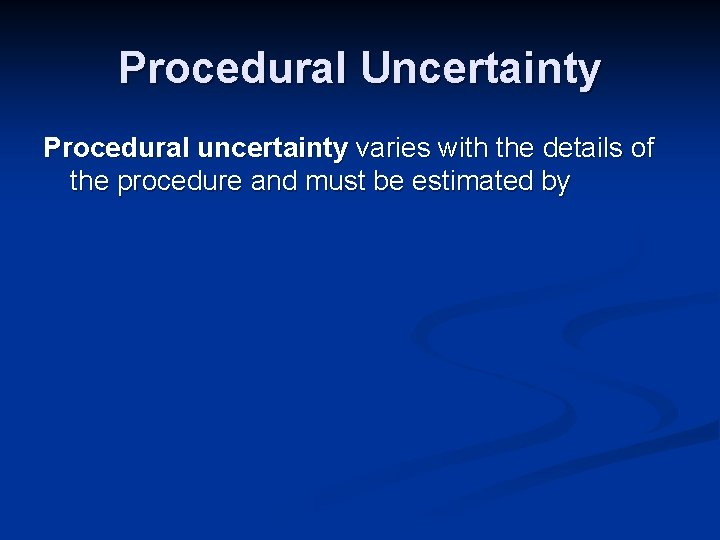Procedural Uncertainty Procedural uncertainty varies with the details of the procedure and must be estimated byProcedural Uncertainty Procedural uncertainty varies with the details of the procedure and must be estimated by the experimenter.Procedural Uncertainty Procedural uncertainty varies with the details of the procedure and must be estimated by the experimenter. For example, if a 30 -cm ruler were used to measure the length of a field by lifting with ruler and placing it back down repeatedly, an uncertainty associated with the repositioning of the ruler is introduced and may be estimated by the experimenter at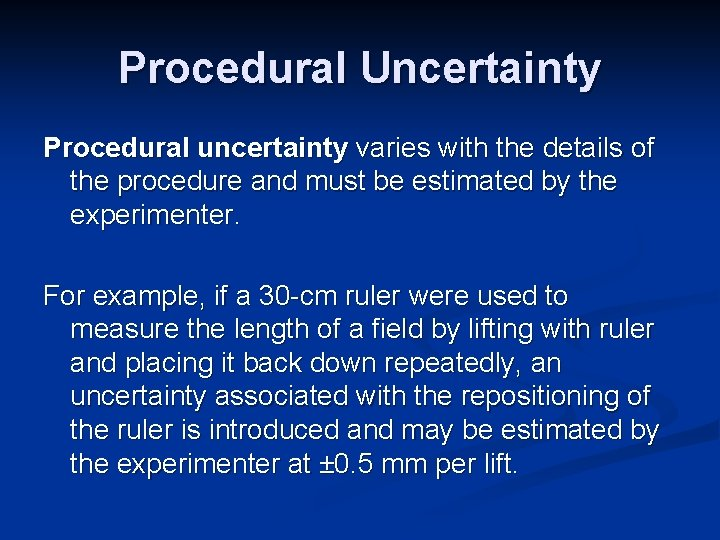Procedural Uncertainty Procedural uncertainty varies with the details of the procedure and must be estimated by the experimenter. For example, if a 30 -cm ruler were used to measure the length of a field by lifting with ruler and placing it back down repeatedly, an uncertainty associated with the repositioning of the ruler is introduced and may be estimated by the experimenter at ± 0. 5 mm per lift.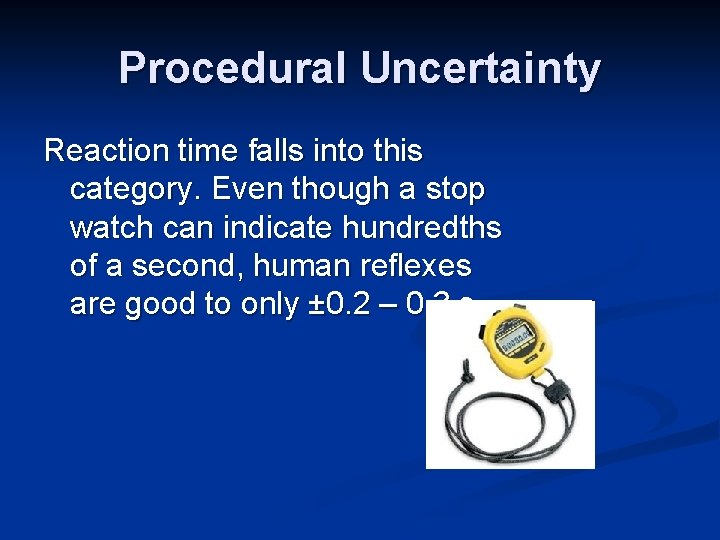Procedural Uncertainty Reaction time falls into this category. Even though a stop watch can indicate hundredths of a second, human reflexes are good to only ± 0. 2 – 0. 3 s.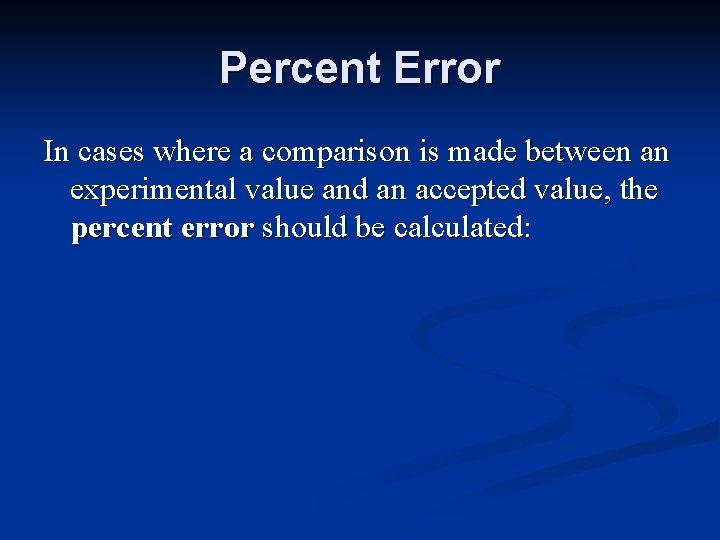Percent Error In cases where a comparison is made between an experimental value and an accepted value, the percent error should be calculated:Percent Error In cases where a comparison is made between an experimental value and an accepted value, the percent error should be calculated: (written as a percent, so x 100)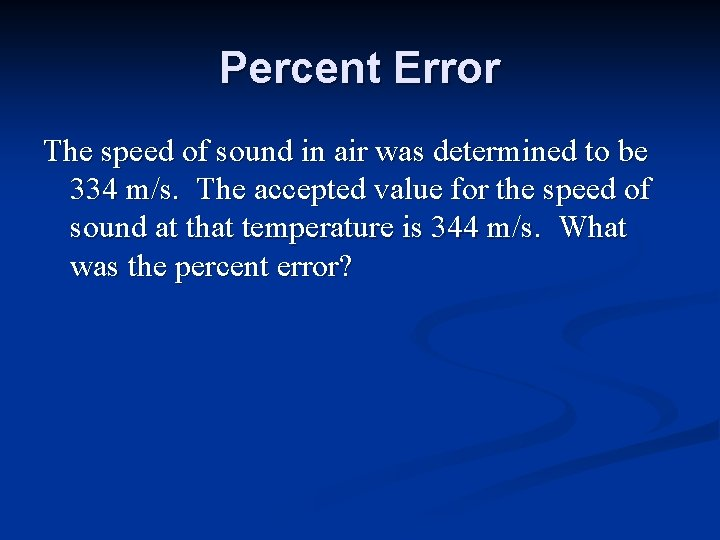Percent Error The speed of sound in air was determined to be 334 m/s. The accepted value for the speed of sound at that temperature is 344 m/s. What was the percent error?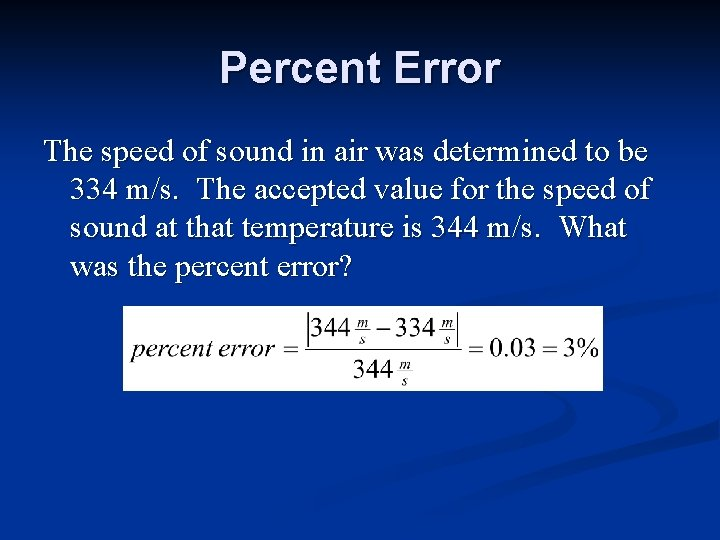Percent Error The speed of sound in air was determined to be 334 m/s. The accepted value for the speed of sound at that temperature is 344 m/s. What was the percent error?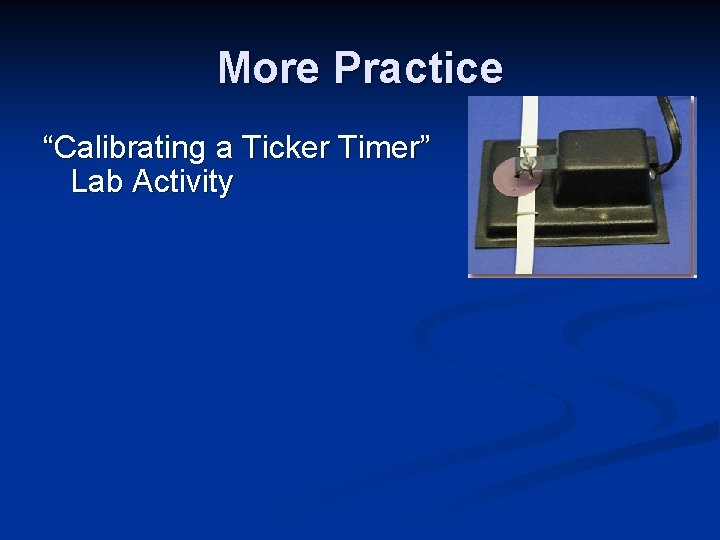More Practice “Calibrating a Ticker Timer” Lab Activity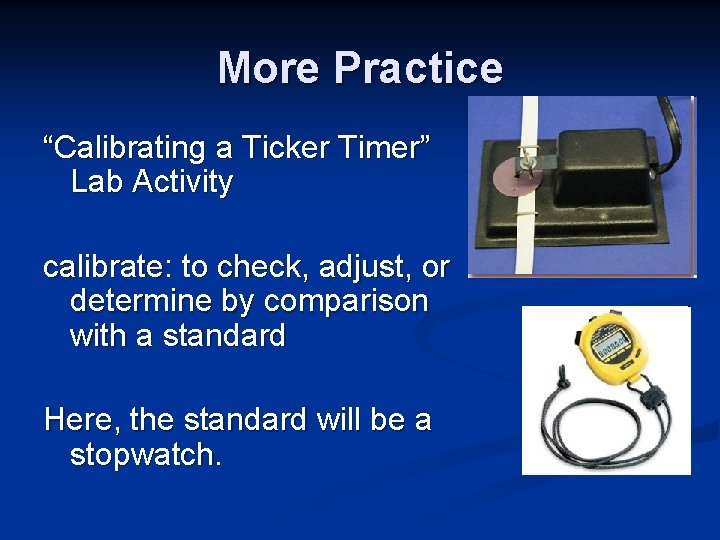More Practice “Calibrating a Ticker Timer” Lab Activity calibrate: to check, adjust, or determine by comparison with a standard Here, the standard will be a stopwatch.